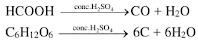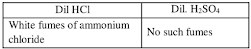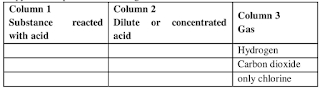# ICSE Solutions for Chapter 11 Sulphuric Acid Class 10 Selina Chemistry

### Exercise

Question 1: Comment, sulphuric acid is referred to as:

(a) King of chemicals
(b) Oil of vitriol

Solution 1: (a) Sulphuric acid is called King of Chemicals because there is no other manufactured compound which is used by such a large number of key industries.

(b) Sulphuric acid is referred to as Oil of vitriol as it was obtained as an oily viscous liquid by heating crystals of green vitriol.

Question 2: Sulphuric acid is manufactured by contact process
(a) Give two balanced equations to obtain SO2 in this process.
(b) Give the conditions for the oxidation of SO2

Solution 2: (a) Two balanced equations to obtain SO2 is:
(i) 4FeS2 + 11O2 → 2Fe2O3 + 8SO2
(ii) S + O2 → SO2

(b) The conditions for the oxidation of SO2 are:
(i) The temperature should be as low as possible. The yield has been found to be maximum at about 410°C – 450°C
(ii) High pressure (2 atm) is favoured because the product formed has less volume than reactant.
(iii) Excess of oxygen increases the production of sulphur trioxide.
(iv) Vanadium pentoxide or platinised asbestos is used as a catalyst.

(c) Sulphuric acid is not obtained directly by reacting SO3 with water because the reaction is
highly exothermic which produce the fine misty droplets of sulphuric acid that is not directly
absorbed by water.

(d) The chemical used to dissolve SO3 is concentrated sulphuric acid. The product formed is oleum.
(e) Main reactions of this process are:
S + O2 → SO3SO3 + H2SO4 → H2S2O7
H2S2O7 + H2O → 2H2SO4

Question 3: Why is water not added to concentrated H2SO4 in order to dilute it?

Solution 3: Water is not added to concentrated acid since it is an exothermic reaction. If water is added to the acid, there is a sudden increase in temperature and the acid being in bulk tends to spurt out with serious consequences.

Question 4: Why the impurity of arsenic oxide must be removed before passing the mixture of SO4 and air through the catalytic chamber?

Solution 4: Impurity of ARSENIC poisons the catalyst [i.e. deactivates the catalyst]. So, it must be removed before passing the mixture of SO2 air through the catalytic chamber.

Question 5: Give two balanced reactions of each type to show the following properties of sulphuric acid:
(a) Acidic nature. (b) The oxidising agent,
(c) Hygroscopic nature, (d) Non-volatile nature

Solution 5: Balanced reactions are:
(a) Acidic nature:
(i) Dilute H2SO4 reacts with basic oxides to form sulphate and water.
2 NaOH + H2SO4 → Na2SO4 + 2H2O
(ii) CuO + H2SO4 →  CuSO4 + H2O
(iii) It reacts with carbonate to produce CO2.
Na2CO3 + H2SO4 → Na2SO4 + H2O + CO2

(b) Oxidising agent:
H2SO4 → H2O + SO2 + [O]
Nascent oxygen oxidizes non-metals, metals and inorganic compounds.
For example,
Carbon to carbon dioxide
C + H2SO4 → CO2 + H2O + 2SO2
Sulphur to sulphur dioxide
S + H2SO4 → 3SO2 + 2H2O

(c) Hygroscopic nature:
It has great affinity for water. It readily absorbs moisture from atmospheric air.(d) Non-volatile nature:

It has a high boiling point (356°C) so it is considered to be non-volatile. Therefore, it is used for preparing volatile acids like hydrochloric acid, nitric acid from their salts by double decomposition reaction.
NaCl + H2SO4 → NaHSO+ HCl
KCl + H2SO4 → KHSO + HCl

Question 6: Give a chemical test to distinguish between:
(a) dilute sulphuric acid and dilute hydrochloric acid
(b) dilute sulphuric acid and conc. Sulphuric acid

Solution 6: (a) Bring a glass rod dipped in Ammonia solution near the mouth of each test tubes containing dil. Hel and dil. H2SO4 each.(b) 1. Dilute sulphuric acid treated with zinc gives Hydrogen gas which bums with pop sound.
Concentrated H2SO4 gives SO2 gas with zinc and the gas turns Acidified potassium
dichromate paper green.

2. Barium chloride solution gives white ppt. with dilute H2SO4. This white ppt. is insoluble in all acids.
Concentrated H2SO4 and NaCl mixture when heated gives dense white fumes if glass rod dipped in Ammonia solution is brought near it.

Question 7: Name the products formed when hot and concentrated sulphuric acid reacts with the following:
(a) Sulphur (b) NaOH,
(c) Sugar (d) Carbon
(e) Copper.

Solution 7: (a) When sulphuric acid reacts with sulphur the product formed is Sulphur dioxide is formed.
S + 2H2SO4 → 3SO2 + 2H2O

(b) When sulphuric acid reacts with sodium hydroxide it neutralizes base to form sodium sulphate.
2NaOH + H2SO4 → Na2SO4 + 2H2O

(c) When sulphuric acid reacts with sugar it forms carbon(d) When sulphuric acid reacts with carbon it forms carbon dioxide and sulphur dioxide gas.
C + 2H2SO4 → CO2 + 2H2O + 2SO2

(e) When sulphuric acid reacts with copper it forms copper sulphate and sulphur dioxide.
Cu + H2SO4 → CuSO4 + 2H2O + SO2

Question 8: Why is: (a) Concentrated sulphuric acid kept in airtight bottles?
(b) H2SO4 not a drying agent for H2S?
(c) Sulphuric acid used in the preparation of HCI and HNO3? Give equations in both cases.

Solution 8: (a) Concentrated sulphuric acid is a hygroscopic substance that absorbs moisture when exposed to air. Hence, it is stored in air tight bottles.

(b) Sulphuric acid is not a drying agent for H2S because it reacts with H2S to form sulphur.
H2SO4 + H2S → 2H2O + SO2 + S↓

(c) Concentrated sulphuric acid has high boiling point (356°C). So, it is considered to be non-volatile. Hence, it is used for preparing volatile acids like Hydrochloric acid and Nitric acids from their salts by double decomposition.
NaCl + H2SO4 → NaHSO4 + HCl
NaNO3 + H2SO4 → NaHSO4 + HNO3

Question 9: What property of conc. H2SO4 is made use of in each of in each of the following cases? Give an equation for the reaction on each case:
(a) in the production of HCI gas when it reacts with a chloride,
(b) in the preparation of CO and HCOOH,
(c) as a source of hydrogen by diluting it and adding a strip of magnesium.
(d) in the preparation of sulphur dioxide by warming a mixture of conc. Sulphuric acid and copper – turnings,
(e) Hydrogen sulphide gas is passed through concentrated sulphuric acid.

Solution 9: (a) Due to its reducing property. i.e, it is a non-volatile acid.
NaCl + H2SO4 → NaHSO4 + HCI
(Conc.)

(b) It is a dehydrating agent.

(c) Magnesium is present above hydrogen in the reactivity series so sulphuric acid is able to liberate hydrogen gas by reacting with a magnesium strip.
Mg + H2SO4 → MgSO4 + H

(d) Due to its oxidizing character
Cu + H2SO4 → CuSO4 + 2H2O + SO

(e) Due to its oxidizing property Hydrogen sulphide gas is passed through concentrated sulphuric acid to liberate sulphur dioxide and sulphur is formed.
H2S + H2SO4 → S + 2H2O + SO2

Question 10: What is the name given to the salts of:
(a) sulphurous acid (b) sulphuric acid?

Solution 10: The name of the salt of
(a) Hydrogen sulphites and Sulphites.
(b) Sulphate and bisulphate.

Question 11: Give reasons for the following:
(a) Sulphuric acid forms two types of salts with NaOH,
(b) Red-brown vapours are produced when concentrated sulphuric acid is added to hydrogen bromide.
(c) A piece of wood becomes black when concentrated sulphuric acid is poured on it,
(d) Brisk effervescence is seen when oil of vitriol is added to sodium carbonate.

Solution 11: (a) Two types of salts are formed when sulphuric acid reacts with NaOH because sulphuric acid is dibasic.
NaOH + H2SO4 → NaHSO4 + H2O
2NaOH + H2SO4 → Na2SO4 + 2H2O

(b) When hydrogen bromide reacts with sulphuric acid the bromine gas is obtained which produce red-brown vapours.
2KBr + 3H2SO+ 2KHSO4 + SO+ Br2 + 2H2O

(c) A piece of wood becomes black when concentrated sulphuric acid is poured on it because it gives a mass of carbon.

(d) When sulphuric acid is added to sodium carbonate it liberates carbon dioxide which produces brisk effervescence.
Na2CO3 + H2SO4 → Na2SO4 + H2O + CO2

Question 12: Copy and complete the following table:Solution 12:
 Column 1 Substance reacted with acid Column 2 Dilute or concentrated acid Column 3 Gas Substance reacted with acid. Dilute or concentrated sulphuric acid. Gas Zinc Dilute sulphuric acid Hydrogen Calcium carbonate Concentrated sulphuric acid Carbon dioxide Bleaching powder (CaOCl2) Dilute sulphuric acid Only chlorine

Question 13: Name a gas that can be oxidized to sulphur.

Solution 13: Hydrogen sulphide (H2S) can be oxidized to sulphur.

Question 14: Give the odour of the gas evolved and name the gas produced when sodium sulphide is added to solution of HCI in water.

Solution 14: When sodium sulphide is added to solution of HCl, Hydrogen sulphide gas is produced. It has rotten egg like smell.

Question 15:(a) Name the catalyst which helps in the conversion of sulphur dioxide to sulphur trioxide in step C.
(b) In the contact process for the manufacture of sulphuric acid, sulphur trioxide is not converted to sulphuric acid by reacting it with water. Instead a two-steps procedure is used. Write the equations for the two steps involved in D.
(c) What type of substance will liberate sulphur dioxide from sodium sulphite in step E?
(d) Write the equation for the reaction by which sulphure dioxide is converted to sodium
sulphite in step F.

Solution 15: (a) The catalyst which helps in the conversion of sulphur dioxide to sulphur trioxide in step C is Vanadium pentoxide.

(b) The two steps for the conversion of sulphur trioxide to sulphuric acid is:
(i) SO3 + H2SO4 → H2S2O
(ii) H2S2O7 + H2O → 2H2SO4

(c) The substance that will liberate sulphur dioxide in step E is dilute H2SO4.

(d) The equation for the reaction by which sulphur dioxide is converted to sodium sulphite in step F is:
SO2 + 2NaOH → Na2SO3 + H2O
Na2O + SO2 → Na2SO3

Question 16: (a) Name the process used for the large-scale manufacture of sulphuric acid.
(b) Which property of sulphuric acid accounts for its use as a dehydrating agent?
(c) Concentrated sulphuric acid is both an oxidizing agent and a non-volatile acid. Write one equation each to illustrate the above-mentioned properties of sulphuric acid.

Solution 16: a) The process used for the large-scale manufacture of sulphuric acid is Contact process.

(b) Sulphuric acid has great affinity for water. It readily removes element of water from other compound. Thus it acts as a dehydrating agent.

(c) Concentrated acid is non-volatile thus it is used for the preparation of volatile acids:
NaCl + H2SO4 → NaHSO4 + HCI
Concentrated acid act as an oxidizing agent:
C + 2H2SO4 → CO2 + 2H2O + 2SO2

Question 17: Some properties of sulphuric acid are listed below. Choose the property A, B, C or D which is responsible for the reactions (i) to (v) some properties may be repeated.
A. Acid
B. Dehydrating agent
C. Non-volatile acid
D. Oxidizing agent
(i) C12H22O11 + NH2SO4 → 12C + 11H2O + nH2SO4
(ii) S + 2H2SO4 → 3SO2 + 2H2
(iii) NaCl + H2SO4 → NaHSO4 + HCI
(iv) CuO + H2SO4 → CuSO+ H2
(v) Na2CO3 + H2SO4 → Na2SO4 + H2O + CO2

Solution 17:
(i) B
(ii) D
(iii) C
(iv) A
(v) A

Question 18: (a) Name the acid formed when sulphur dioxide dissolves in water
(b) Name the gas released when sodium carbonate is added to a solution of sulphur dioxide.

Solution 18: (a) The acid formed when sulphur dioxide dissolves in water is sulphurous acid.

(b) Carbon dioxide gas is released when sodium carbonate is added to solution of sulphur dioxide.

Question 19: (a) What is the property of concentrated sulphuric acid which allows it to be used in the preparation of hydrogen chloride and nitric acid?
(b) What property of concentrated sulphuric acid is in action when sugar turns black in its presence?

Solution 19: (a) Concentrated sulphuric acid is non-volatile; hence it is used for the preparation of higher volatile acids.

(b) Due to its dehydrating nature sugar turns black in the presence of concentrated sulphuric
acid.